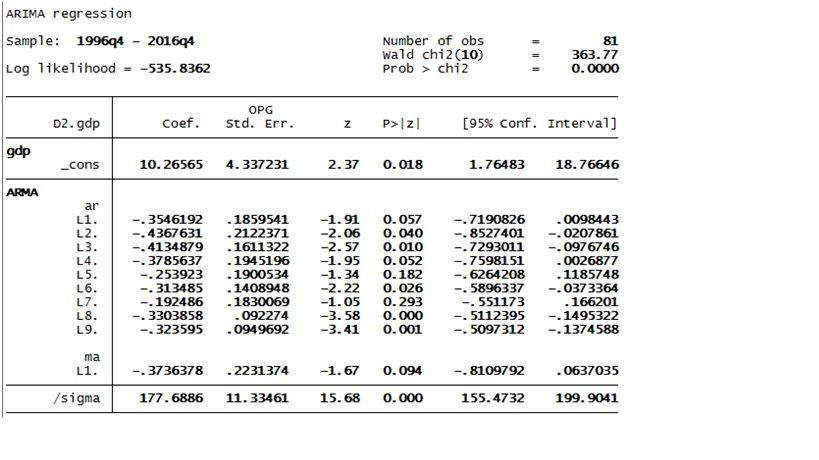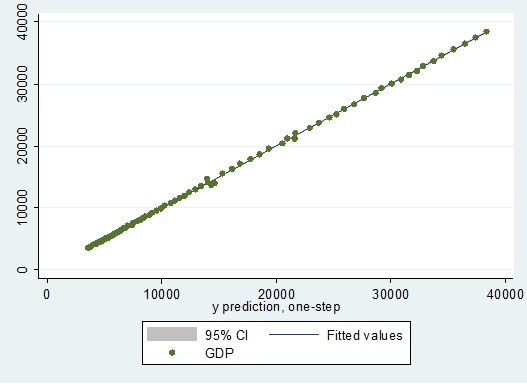# How to predict and forecast using ARIMA in STATA?

After performing Autoregressive Integrated Moving Average (ARIMA) modelling in the previous article: ARIMA modeling for time series analysis in STATA, the time series GDP can be modelled through ARIMA (9, 2, 1) equation as below:Figure 1: ARIMA Results in STATA

ARIMA results as in the above figure can be analysed through several components:

1. Log likelihood: the value of log likelihood is 535.8 which is minimum among all the ARIMA models.
2. AIC & BIC: The value of AIC and BIC is also less than previous model but only up to 1 point.

## Prediction in ARIMA

To generate the prediction use the command:

`STATA Command: predict chat, y`

The commands ‘predict’ is used for generating values based on selected model. In present case this is fixed effect model. Furthermore, ‘chat’ is the term given to the fitted variable of GDP. Lastly, ‘y’ denotes the fitted values. Now a separate variable as ‘chat’ will be created in data editor window. This is the fitted values of GDP. The command for standard errors is:

`STATA Command: predict stdp`

Furthermore, represent the fitted and actual values together in two way graph. It shows standard deviations as scattered around the fitted line. For that use the command:

`STATA Command: twoway lfitci  gdp chat, stdp || scatter  gdp chat`

Here,

• ‘twoway’ is for two way graphs in STATA
• ‘lfitci’ stands for line of best fit with confidence interval
• ‘gdp’ is actual variable
• ‘chat’ is predicted variable
• ‘Stdp’ is estimated standard error
• ‘Scatter’ means scatter plot.

After running the command, the below graph will appear. It shows green dots as actual GDP values, shaded region as confidence interval and straight line as fitted values.Figure 2: Two way graph of actual and fitted values of GDP

The above graph shows that the fitted values fully take into account the scattered values of GDP. That means although the mean of both the series are same, ‘predict’ values of GDP do not differ from actual values of GDP.

## Forecasting

To generate the prediction use the command:

`STATA Command: predict chatdy, dynamic(tq(2017q1)) y`

Here,

The command ‘predict’ is used for generating values based on selected model. In the present case is fixed effect model.  Furthermore,‘chatdy’ is the name for the forecasted variable of GDP. Lastly, ‘dynamic’ denotes the dynamic forecasting of STATA.

Now, a separate variable as chat will appear in data editor window. This shows the forecasted values of GDP. The forecasted values of GDP for 2017 are:

 Time Period GDP Forecasts 2017 Q1 39333.45 2017 Q2 40220.4 2017 Q3 41081.47 2017 Q4 42014.04

Thus, ARIMA equations are useful in forecasting the values of GDP. However, ARIMA is insufficient is defining the econometrics model with more than one variable. Therefore, multi-variate time series is necessary in some cases. The proceeding articles explain the procedure for conducting Vector Auto-Regression (VAR) analysis, which is a popular method of multi-variate time series.

### Priya Chetty

Partner at Project Guru
Priya is a master in business administration with majors in marketing and finance. She is fluent with data modelling, time series analysis, various regression models, forecasting and interpretation of the data. She has assisted data scientists, corporates, scholars in the field of finance, banking, economics and marketing.

### Related articles

• VECM in STATA for two cointegrating equations Unrestricted Vector Auto Regression (VAR) is not applicable in such cases. Vector Error Correction Model (VECM) is a special case of VAR which takes into account the cointegrating relations among the variables.
• ARIMA modeling for time series analysis in STATA In the previous article, all possibilities for performing Autoregressive Integrated Moving Average (ARIMA) modeling for the time series GDP were identified as under. S. […]
• How to perform Johansen cointegration test in VAR with three variables? The previous article showed lag selection and stationarity for Vector Auto Regression (VAR) with three variables; Gross Domestic Product (GDP), Gross Fixed Capital Formation (GFC) and Private Final Consumption (PFC). This article shows the co-integration test for VAR with three variables.
• Lag selection and cointegration test in VAR with two variables The previous article showed that the three-time series values Gross Domestic Product (GDP), Gross Fixed Capital Formation (GFC) and Private Final Consumption (PFC) are non-stationary. Therefore they may have long-term causality. The general assumption, in this case, is that consumption […]
• Building univariate ARIMA model for time series analysis in STATA Autoregressive Integrated Moving Average (ARIMA) is popularly known as Box-Jenkins method. The emphasis of this method is on analyzing the probabilistic or stochastic properties of a single time series. Unlike regression models where Y is explained by X1 X2….XN regressor (like […]We are looking for candidates who have completed their master's degree or Ph.D. Click here to know more about our vacancies.